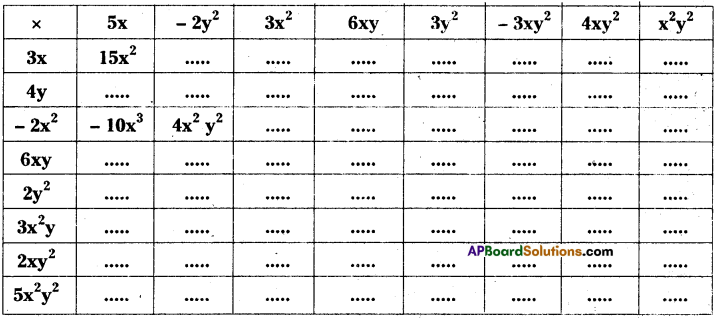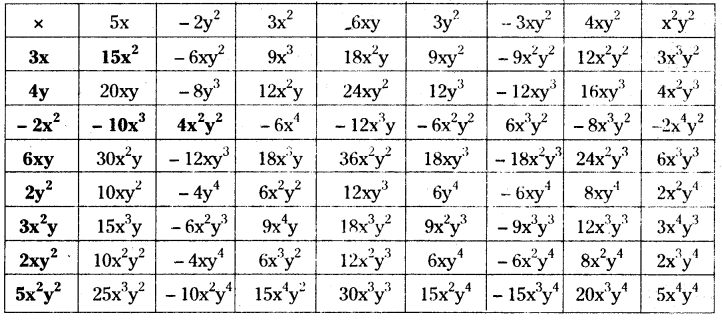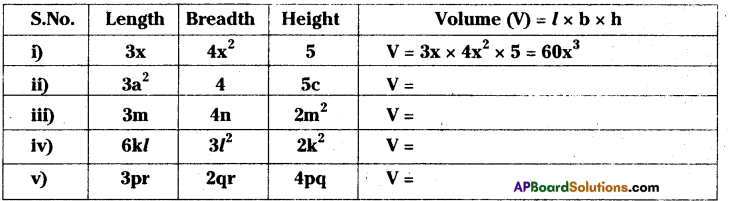AP State Syllabus AP Board 8th Class Maths Solutions Chapter 11 Algebraic Expressions Ex 11.1 Textbook Questions and Answers.

## AP State Syllabus 8th Class Maths Solutions 11th Lesson Algebraic Expressions Exercise 11.1Question 1.
Find the product of the following pairs:
(i) 6, 7k
(ii) – 31, – 2m
(iii) -5t2 – 3t2
(iv) 6n, 3m
(v) – 5p2, – 2p
Solution:
The product of 6, 7k = 6 × 7k = 42k
ii) The product of – 3l, – 2m = (- 3l) × (- 2m) = 6/m
iii) The product of – 5t2, – 3t2 = (- 5t2) × (- 3t2) = 15t4
iv) The product of 6n, 3m = 6n × 3m = 18mn
v) The product of – 5p2, – 2p = (- 5p2) × (- 2p) = 10p3Question 2.
Complete the table of the products.Solution:Question 3.
Find the volumes of rectangular boxes with given length, breadth and height in the following table.Solution:Question 4.
Find the product of the following monomials
(i) xy, x2y , xy, x
(ii) a, b, ab, a3 b, ab3
(iii) kl, lm, km, klm
(iv) pq ,pqr, r
(v) – 3a, 4ab, – 6c, d
Solution:
i) The product of xy, x2y, xy, x = xy × x2y × xy × x
= x5 × y3= x5y3

ii) The product of a, b, ab, a3b, ab3 = a × b × ab × a3b × ab3
= a6 × b6 = a6 b6

iii) The product of kl, lm, km, klm = kl × lm × km × klm
k3 × l3 × m3 =k3l3m3

iv) The product of pq, pqr, r = pq × pqr × r
= p2 × q2 × r2 – p2q2r2

v) The product of – 3a, 4ab, – 6c, d = (- 3a) × 4ab × (- 6c) x d
= + 72a2 × b × c × d
= 72a2bcd

Question 5.
If A = xy,B = yz and C = zx, then find ABC=
Solution:
ABC = xy × yz × zx = x2y2z2Question 6.
If P = 4x2, T = 5x and R = 5y, then $$\frac{\mathrm{PTR}}{100}$$ =
Solution:
$$\frac{P^{\prime} \Gamma R}{100}=\frac{4 x^{2} \times 5 x \times 5 y}{100}=\frac{100 x^{3} y}{100}$$ = x3 y

Question 7.
Write some monomials of your own and find their products.
Solution:
The product of,some monomials is given below :
i) abc × a2bc = a3b2c2
ii) xy × x2z × yz2 = x3y2z3
iii) p × q × r = p3q3r3